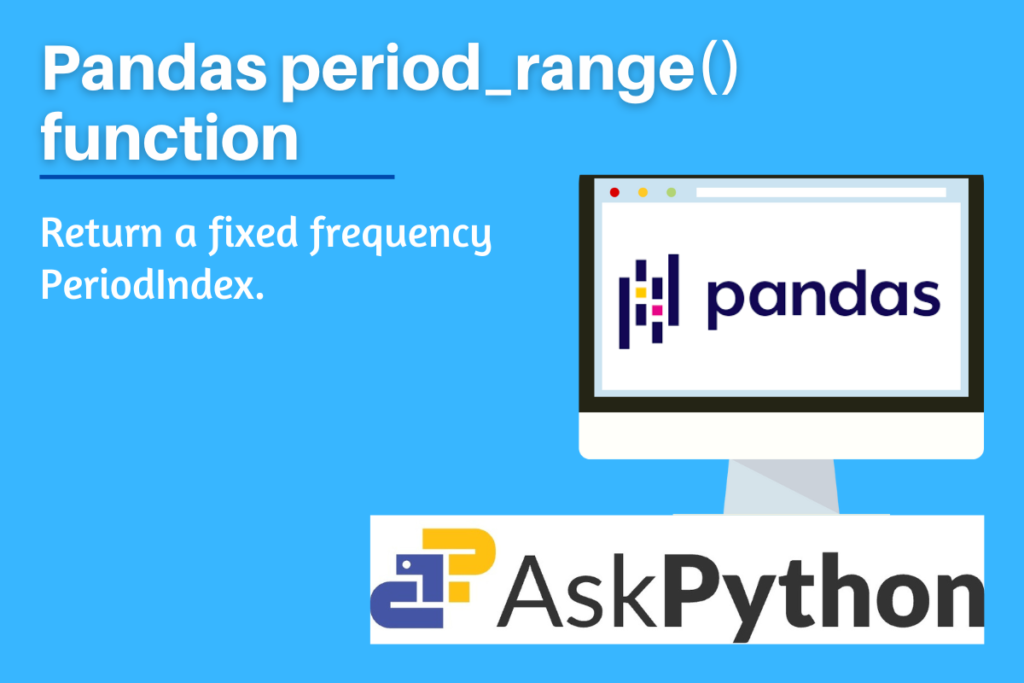# Pandas period_range – Return a fixed frequency PeriodIndexIn this post, let us try to understand the Pandas period_range() function, one of the Pandas package’s general methods. The Pandas package for Python language is used for data processing and comprehension. Both “Panel Data” and “Python Data Analysis” are intended to be referred to as “Pandas.” It facilitates working with time series and mathematical as well as statistical data tables by providing specific methodologies, built-in functions, and data types. Pandas is an open-source package and one of the best tools to deal with real-world messy and unorganized data. It is the top priority for data scientists and data analysts.

period_range() is one such built-in function of the Pandas package which is commonly used to return a fixed frequency output. Let us try to understand its use cases, syntax, and implementation in the Python Language.

Also read: What is Pandas bdate_range() function

## What is the need for the Pandas period_range() function?

This function is used to return a PeriodIndex with a set frequency. An immutable n-dimensional array called PeriodIndex contains ordinal values that represent regular time periods. It is necessary to specify exactly two out of the three parameters (start, end, and periods). The default frequency is based on the day (calendar).

Also, check out the timedelta_range() function of the Pandas package. It is very similar to the function we are discussing. The only difference is that it returns a fixed frequency in TimedeltaIndex format.

## Syntax of Pandas period_range

```pandas.period_range(start=None, end=None, periods=None, freq=None, name=None)
```
• start: string or period-like, default is set to None
• This value is used as a left bound.
• end: string or period-like, default is set to None
• This value is used as a right bound.
• periods: integer, default is set to None
• The number of periods to produce.
• freq: string or DateOffset, optional
• alias for frequency If start or end are Period objects, the frequency is by default taken from those items. In all other cases, the daily frequency setting is “D”.
• name: string, default is set to None, optional
• Name of the resulting PeriodIndex

## Implementing Pandas period_range

Before beginning the methods, be sure to load the pandas package into your IDE. Run the following line of code in your IDE to accomplish this.

```import pandas as pd
```

### Example 1: Passing only start and end parameters only

```pd.period_range(start='2023-01-01', end='2023-01-05')
```

Output

Note when a ‘periods’ or frequency – ‘freq’ parameters are not passed, then the default frequency will be ‘day’, and the output will include every day between the start and end day.

### Example 2: Passing frequency parameter

```#frequency set to week, will display start and end day of the week.
pd.period_range(start='2023-01-01', end='2023-02-01', freq='W')
```

Output

Note that in the above example, the frequency parameter is set to ‘W’ which stands for a week. Hence in the output, each value has two dates, the first date indicates the first day of the week which is Monday and the other one indicates the last day of that week which is Sunday.

### Example 3: Passing periods parameter

```pd.period_range(start='2023-01-01', periods=5, freq='M')

pd.period_range(start='2023-01-01', periods=3, freq='Y', name='outcome')
```

Output